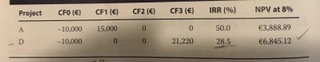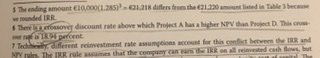# IRR example page 219 Vol 1

Hi all, I understand the interpretation of IRR on page 219 of Reading 6, Vol 1. However, it says this on the 5th line in the first paragraph (after the example) - " If we subtract a capital charge of Y270,570,310 (the amount of a 5-year annuity having a PV of Y1,000 million at 11%)…" How did it arrived at the figure of Y270,570,310? Is it using FV = A [(1- (1 + R)^n) / r] ? if so i cannot get it to the Y270,570,310 having A = Y1,000 million (as I’m thinking you’d want the FV of the capital charge???) Please help, trying to digest this bit

1 Like

Terminology based on TI BA II Plus calc: Do PV = -1000 N=5 I/Y=11 CPT PMT Gives you 270.570310

hello,

thank you so much for the explanation.

There’s another example in very next section (table 3) where two projects are compared.I would like to understand how a crossover discount rate was calculated for following note #6:Best,
Gaurav

Take the difference in the project cash flows, say cashflow of project A minus cashflow of project D:

T = 0: -10,000 - (-10,000) = 0
T = 1: 15,000 - 0 = 15,000
T = 2: 0 - 0 = 0
T = 3: 0 - 21,200 = -21,200

Calculate the IRR of the difference in the cash flows and you will get the crossover rate.

1 Like